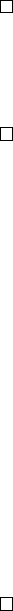4Quotient groups

IA Groups4.1 Normal subgroups
Definition (Normal subgroup). A subgroup K of G is a normal subgroup if
(a G)(k K) aka
1
K.
We write K C G. This is equivalent to:
(i) (a G) aK = Ka, i.e. left coset = right coset
(ii) (a G) aKa
1
= K (cf. conjugacy classes)
From the example last time,
H
=
hsi D
6
is not a normal subgroup, but
K
=
hri C D
6
. We know that every group
G
has at least two normal subgroups
{e} and G.
Lemma.
(i) Every subgroup of index 2 is normal.
(ii) Any subgroup of an abelian group is normal.
Proof.
(i)
If
K G
has index 2, then there are only two possible cosets
K
and
G \K
.
As
eK
=
Ke
and cosets partition
G
, the other left coset and right coset
must be G \ K. So all left cosets and right cosets are the same.
(ii) For all a G and k K, we have aka
1
= aa
1
k = k K.
Proposition. Every kernel is a normal subgroup.
Proof.
Given homomorphism
f
:
G H
and some
a G
, for all
k ker f
, we
have
f
(
aka
1
) =
f
(
a
)
f
(
k
)
f
(
a
)
1
=
f
(
a
)
ef
(
a
)
1
=
e
. Therefore
aka
1
ker f
by definition of the kernel.
In fact, we will see in the next section that all normal subgroups are kernels
of some homomorphism.
Example.
Consider
G
=
D
8
. Let
K
=
hr
2
i
is normal. Check: Any element of
G
is either
sr
`
or
r
`
for some
`
. Clearly
e
satisfies
aka
1
K
. Now check
r
2
:
For the case of
sr
`
, we have
sr
`
r
2
(
sr
`
)
1
=
sr
`
r
2
r
`
s
1
=
sr
2
s
=
ssr
2
=
r
2
.
For the case of r
`
, r
`
r
2
r
`
= r
2
.
Proposition. A group of order 6 is either cyclic or dihedral (i.e.
=
C
6
or D
6
).
Proof.
Let
|G|
= 6. By Lagrange theorem, possible element orders are 1
,
2
,
3 and
6. If there is an
a G
of order 6, then
G
=
hai
=
C
6
. Otherwise, we can only
have elements of orders 2 and 3 other than the identity. If
G
only has elements
of order 2, the order must be a power of 2 by Sheet 1 Q. 8, which is not the case.
So there must be an element
r
of order 3. So
hri C G
as it has index 2. Now
G
must also have an element s of order 2 by Sheet 1 Q. 9.
Since
hri
is normal, we know that
srs
1
hri
. If
srs
1
=
e
, then
r
=
e
,
which is not true. If
srs
1
=
r
, then
sr
=
rs
and
sr
has order 6 (lcm of the
orders of
s
and
r
), which was ruled out above. Otherwise if
srs
1
=
r
2
=
r
1
,
then G is dihedral by definition of the dihedral group.